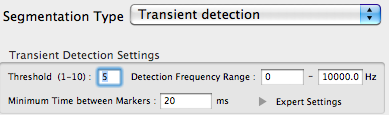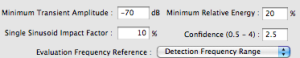IntroductionSignal Analysis > Markers > Automatic Markers > Transients Detection > Transient Detection Settings
page précédentepage suivante

# Transient Detection Settings

## Basic SettingsThe basic settings of the transient detection include a detection threshold, a frequency range and a time between markers.

Window Size and Analysis Accuracy

Although the window size is not included in the detection settings, it has an impact over the sensitivity of the transient detector.

A large window size with a high frequency resolution improve the segregation of attack transients and stationary sinusoids.

A small window size allows to detect more than one attack transient.

Detection Threshold

A ratio from 1 to 10.

This ratio is calculated from the relation between the global signal energy and the transient energy, or NEV.

• The lower the ratio, the lower the detection sensibility is. The risk to confuse residual noise with transients is important.

• A ratio of 10 will probably yield no transients.

Detection Frequency Range

Bandwidth in Hz.

This bandwidth can be defined freely by the user. By default, Flow = 0, Fhigh = Samplerate/2 (Nyquist Frequency), which are also the maximum frequency limits.

• The evaluation of the energy variation and statistical model for background transient activity takes place in this frequency band.

• This value is only operative for transient detections. Treatments requiring transients preservation and remix use the whole signal bandwith.

Minimum Time between Markers

A value in ms.

• By default, the time is equal to 20 ms. A too short interval will yield several markers for the same transient.

• A transient is correctly classified if the difference between the estimated start time is not superior to 50ms.

## Expert SettingsThe expert settings allow to refine the analysis parameters, especially as far as the statistical model is concerned. The detection threshold can be expressed as a value in dB or a percentage of energy of the signal. The type of frequency range where the detection is executed can be selected. The bandwidth used to define the statistical model that allows to segregate transients and onsets can also be specified with the single sinusoid impact factorThe reliability of the statistical model is defined with the confidence ratio.
Minimum Transient Amplitude and Minimum Relative Energy

Detection threshold in dB.

By default, this threshold is equal to -120. The closer we get to 0, the more transients are filtered.

The threshold can also be expressed as a percentage of energy, which is significant from a perceptive point of view. This percentage of energy can be calculated from a given type of frequency range, via the `Evaluation Frequency Reference`.

Evaluation Frequency Reference
• Statistical bands : The statistical model can operate within frequency bands that will not cover the whole spectrum. The bandwidth is determined by the single sinusoid impact factor.

• Detection frequency range : corresponds to the bandwidth specified by the user in the detection frequency range

• Whole frequency band : Nyquist Frequency

Single Sinusoid Impact Factor
• The single sinusoid impact factor (Is) is the size occupied by a sinusoid in a band, expressed as a percentage. By default, this factor is fixed to 10%, so that we get a number (Ns) of 10 sinusoids per band. A 10% factor is generally a good choice.

Ns=1/Is

For instance, with a 20% impact factor, we get :

Ns=(1*100)/20=5

• The bandwidth depends on the number of bins of the sinusoid. With an N bins sinusoid, and a K impact factor, the size is expressed :

S=(N*100)/ K

For instance, with 10 bins sinusoids and a 20% impact factor, we have 5 sinusoids of 10 bins per band, and a 50 bins bandwidth :

S=(10*100)/20 = 50

The sensitivity of the detector also depends on this factor.

• The narrower the bandwidth, the less peaks we need, and the more sensitive the detector is. The larger the band, the more peaks are needed to trigger the transient detector.

• If the bandwidth of the model matches the bandwidth of the transient peak, we get the best results, but this transient bandwidth can not be known in advance.

• In the presence of noise, a decrease in bandwidth may require a larger number of transient peaks to enable transient detection.

Confidence Level and Detection Threshold

The confidence level determines the reliability of the detection when comparing the statistical model and the current frames.

Minimum and maximum values : 0,5-4.

Default value : 2,5.

• A high confidence level means that many simultaneous transient peaks are requested for a transient to be detected : the detector is not very sensitive.

• A low confidence level means that few peaks are requested, and the detector is highly sensitive.

• In the presence of stationary sinusoids, the value of the confidence level can be increased.

• In the presence of noise, it should be decreased.

Generally an increase of the confidence level can be compensated by decreasing the transient detection threshold.

1. NEV : Normalized Energy Variation

Maximum of the ratio between total signal energy and the transient energy over the whole duration of the onset.

page précédentepage suivante